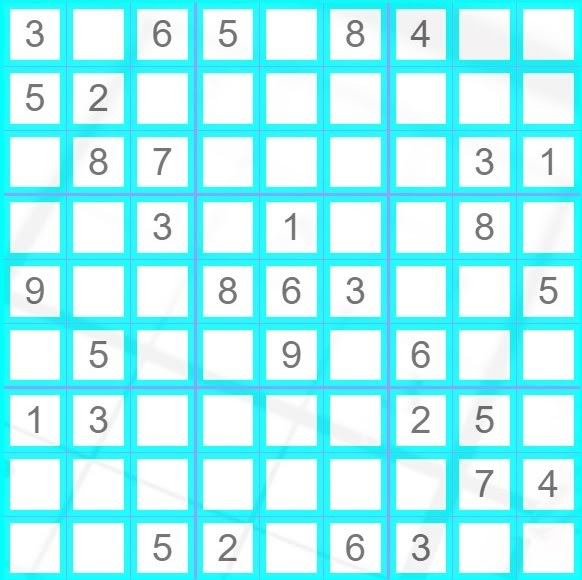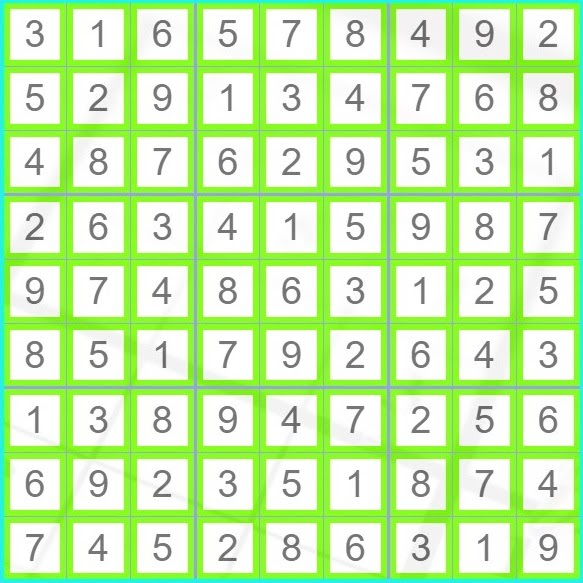# Sudoku Solver

## Introduction

In this article, we will discuss the Sudoku Solver problem with the help of various examples and explanations. The sudoku solver problem is considered a challenging problem and can be of two variations. One variation can be when we have been given unsolved sudoku, and we are required to solve it by filling the blank spaces with the correct number. The other variation is when we have been given unsolved sudoku, and we are supposed to check whether the given numbers are positioned correctly or not. We shall discuss the first variation in this article. The second variation can be found here

Sudoku is a 9x9 puzzle where the board is divided into nine rows and nine columns. It is further divided into nine small grids of 3x3 size each. An element in sudoku is present exclusively in a row or a column, or a grid.

The solution code provided in this article uses the concepts of the Backtracking Algorithm. Readers without prior knowledge might refer to this article- Recursion and Backtracking Algorithm With Practice Problems - CN Blogs before proceeding forward.

### Problem Statement

Write a program to solve the Sudoku puzzle by filling the empty cells on the given board.

Your sudoku solution must satisfy the following rules:

1. Each of the digits 1-9 must occur exactly once in each row.
2. Each of the digits 1-9 must occur exactly once in each column.
3. Each of the digits 1-9 must occur exactly once in each of the 9 3x3 sub-boxes of the grid.

The '.' character indicates empty cells.

### Example and Explanation

Let us use an example to understand better.

Let us consider an input sudoku board that is a vector of vectors of characters. The input is given as:

INPUT

{'3','.','6','5', '.', '8', '4', '.', '.'},

{'5','2','.','.', '.', '.', '.', '.', '.'},

{'.','8','7','.', '.', '.', '.', '3', '1'},

{'.','.','3','.', '1', '.', '.', '8', '.'},

{'9','.','.','8', '6', '3', '.', '.', '5'},

{'.','5','.','.', '9', '.', '6', '.', '.'},

{'1','3','.','.', '.', '.', '2', '5', '.'},

{'.','.','.','.', '.', '.', '.', '7', '4'},

{'.','.','5','2', '.', '6', '3', '.', '.'}

The sudoku formed will look like this:(source: Sudoku Solver Visualizer)

The input is a 9x9 vector of vectors of characters where '.' denotes a blank box. Wherever we encounter a dot, we are supposed to fill a number such that it is unique in its row, column and grid.

On solving the above sudoku, our output must be-

OUTPUT

3 1 6 5 7 8 4 9 2

5 2 9 1 3 4 7 6 8

4 8 7 6 2 9 5 3 1

2 6 3 4 1 5 9 8 7

9 7 4 8 6 3 1 2 5

8 5 1 7 9 2 6 4 3

1 3 8 9 4 7 2 5 6

6 9 2 3 5 1 8 7 4

7 4 5 2 8 6 3 1 9

For better visualization, the output will look like this:(source: Sudoku Solver Visualizer)

## Approach

To solve the problem, we will be using the backtracking algorithm. We will traverse throughout the given sudoku and place a number that falls within the given constraints. If we cannot fill a number at a later stage, it means that we need to go back a few steps and make changes. How does this happen, you may ask? It is possible with the help of backtracking. We undo all the changes made up to the current cell, backtrack to the cell causing the problem, and then start again. We do this until we reach the desired solution. To carry out all the operations successfully, we will be performing the following steps:

• We first create a map that stores in which grid we are and which element are we looking for. For example,

map <pair<int,int>, map<int,int>> mp;

This statement creates a map variable named mp. The first part stores the sudoku grid, like (0,0) or (0,1) and so on. The second part stores the number in the cell of the sudoku.

• We also create two vectors to store the values of rows and columns.
• We then populate our map by using two for loops and checking for '.'. If there is a dot in a cell, we don’t do anything. We change the value at the grid, row, and column to 1 (true). This shows that a particular value at (i,j) in the sudoku board is present in the map at the (i/3, j/3) grid.
• After populating our map and two vectors, we call our recursive function to fill our map and solve the sudoku.
• The name of our recursive function is helper(). It takes six arguments- the row number, the column number, the input board, the map, the row vector, and the column vector.
• It should be noted that we are using pass-by-reference to pass the function arguments. This helps us reduce our space usage drastically.
• The base case of our recursive function is when the row counter reaches 9. This means that we have successfully traversed the whole sudoku board and filled all the values, and we cannot move any further as a sudoku board has only nine rows (0 to 8) and nine columns (0 to 8). So at this stage, we just print our sudoku board.
• In the next condition, we check when the column counter value is 9. This means that the whole column has been traversed, and we have to go to the next row.
• Since we cannot change the already filled cells, we check for such cases and simply move ahead.
• Now comes the main part of our program, where we fill the whole map and both the vectors. We make a for loop starting from 1 up to 9 (the possible numbers that can be placed in our sudoku). Then, for each i, we check if it is present in a particular grid, row, or column. To find out the grid coordinate, we maintain two variables-
• block_row, which is equal to row/3.
• block_column, which is equal to column/3.

These two values help us find the grid. The grid numbering starts from (0,0) in the top left and goes up to (2,2) in the bottom right.

• If a value is not present in a grid, row, or column, we add it to our board and make the corresponding value true in our map, row vector, and column vector. Since our input board is a vector of vectors of characters, we add '0' to the number to convert the number into a character.
• After adding the value to our board, we proceed to the next column by calling the recursive function at (col+1).
• In the solution code provided, we have four extra lines written after the recursive function calling. They are-

mp[{block_row, block_col}][i] = 0;

rows[row][i] = 0;

cols[col][i] = 0;

board[row][col] = '.';

These four lines help us in backtracking. Why do we write it, you may ask? The answer to this is simple. Once a value has been added to our sudoku board, we are not 100% sure that the value inserted by us is correct. At the time of filling that element, it might fulfill all our conditions, but a time may come at a later stage when we are unable to fill our sudoku with an appropriate number. In such cases, we undo all the changes made up until that cell by changing the values in our map and row and column vector to 0 (false). We also undo the change made to our board. These four lines are the most important as they ensure we can fill our sudoku board.

You may be a bit confused after reading the approach. But don’t worry, simplified pseudocode is provided below.

### Pseudocode

1. Start from (0,0) and traverse up to (8,8).
2. Try all the possible valid combinations.
3. If (number already present), then move forward.
4. Else
• for(numbers= 1 to 9)
• If we can place the number at (x,y), then place the number at (x,y) and move forward.

It is highly recommended to write your pseudocode and a partial code before moving to the solution code.

If you are confident enough and want to try out your implement somewhere, don’t worry. You can try the question first on our CodeStudio by clicking here, Sudoku Solver

Output

## Complexities

Time Complexity

In the code mentioned above, we traverse the whole sudoku board and fill all the empty cells. Thus time complexity is,

T(n) = O(9(n*n))

where n is the number of empty cells to be filled

Space Complexity

In the given code, we create a n*n vector to store our sudoku board. The various changes and updates are made to it. Thus,

Space complexity = O(n*n),

where n is the size of the input vector

Q1.) What are the various techniques to solve the Sudoku Solver problem?

Ans.) One of the techniques used to solve the sudoku solver is given in this article. A few other techniques are-

1. Eliminating squares using Naked Pairs in a grid.
2. Eliminating squares using Naked Pairs in rows and columns.
3. Eliminating squares using Hidden Pairs in rows and columns.

## Key Takeaways

To summarize the article, we had an in-depth discussion on the Sudoku Solver problem. We first learned why we should study this problem. After seeing the problem statement and an example, we came up with an approach using backtracking and saw a code for the same.

Learn more about Data Structures and Algorithms through this course: Basics of C++ with Data Structures and Algorithms.

Want to ace the coding rounds of big tech companies? Try our Attempt Unlimited Online Mock Test Series to start your preparation.

Happy Learning!